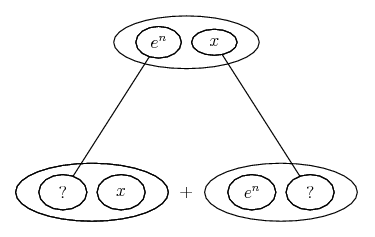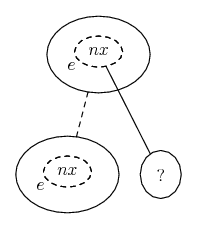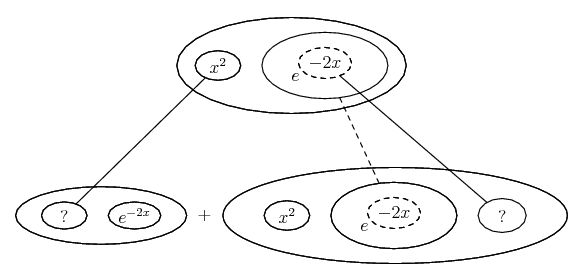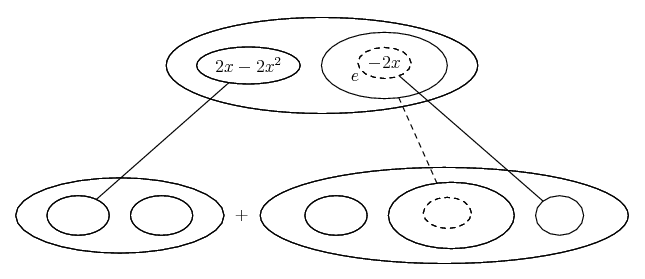# Thread: differential questions.

1. ## differential questions.

Solve the following: Let f(x) = e^nx, where n is a positive integer. Find f ' (x). (Hint: use the Product Rule.)

Let f(x) = x^2/e^2x. Solve f ' ' (x) = 0

I have no idea on how to go about solving those. any help would be appreciated.

2.Originally Posted by nl49Solve the following: Let f(x) = e^nx, where n is a positive integer. Find f ' (x). (Hint: use the Product Rule.)

Let f(x) = x^2/e^2x. Solve f ' ' (x) = 0

I have no idea on how to go about solving those. any help would be appreciated.
$\displaystyle f(x)= x^2e^{2x}= x^2e^{-2x}$. Now, as the hint says, use the product rule. Do you know the derivative of $\displaystyle x^2$? Do you know the derivative of $\displaystyle e^{-2x}$?

3.Originally Posted by nl49Solve the following: Let f(x) = e^nx, where n is a positive integer. Find f ' (x). (Hint: use the Product Rule.)

Let f(x) = x^2/e^2x. Solve f ' ' (x) = 0

I have no idea on how to go about solving those. any help would be appreciated.
I assume that the function is

$\displaystyle f(x) = e^{nx}$

If so you have to use the chainrule:

Let u = nx and u' = n. The derivative of f(x) = e^x is f'(x) = e^x.

Then $\displaystyle f(x)= e^u~\implies~f'(x)=e^u \cdot u'$.

Therefore:

$\displaystyle f'(x) = e^{nx} \cdot n$

4. Just in case a picture helps...... if your product rule hint was in the right place, or..... if not, as earboth suspects. And the other one differentiated once...... and once again...... where...... is the chain rule, and...... the product rule. Straight continuous lines differentiate downwards (integrate up) with respect to x, and the straight dashed line similarly but with respect to the dashed balloon expression (the inner function of the composite which subject to the chain rule).

__________________________________________

Don't integrate - balloontegrate!

Balloon Calculus: Gallery

Balloon Calculus Drawing with LaTeX and Asymptote!

5. I think i may have written the question down wrong. sorry guys.

1.Solve the following: Let f(x) = e^(nx), where n is a positive integer. Find f ' (x). (Hint: use the Product Rule.)

They are two seperate questions.

2.Let f(x) = x^2/e^2x. Solve f ' ' (x) = 0

#### Search Tags

differential, questions